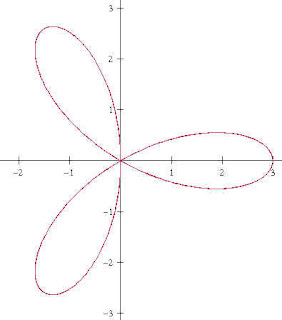## Tuesday, 19 October 2010

### FoliumI realized in Caculus class the other day that I didn't have a link for folium on my Mathwords webpage, so I decided to write one here and just put a link...
--------------------------------------------
Folium is the Latin word for leaf. Student’s frequently are introduced to them in learning to graph polar curves such as r=sin(3*theta) which is a trifoil, or three leaf, sometimes called a “rose” (shown above).

The one that came up in my Calculus class a few days ago was one of the more widely known mathematical folia, the “Folium of Des Cartes”, x3 + y3=3xy. A nice chance to use the newfound skill of implicit differentiation. This curve was created by Des Cartes to challenge the methods of Fermat in finding extrema in the early days of the emerging calculus. I should mention that both men found the extrema without the calculus of limits as we now know it. I mentioned Des Cartes method, as extended by Hudde, in an earlier posting here.The Folium can be rendered in parametric form by using the substitution y=tx to give

x3 + (tx)3 = 3tx2 and dividing out x2 we get to

x + t3 x = 3t... This can be factored for x to get x(1+t3)= 3t.... or

x= (3t) / (1+t3) and thus by y=xt we get y= (3t2)/ (1+t3)

I recently came across this neat stamp image with a picture of Des Cartes and his Folium.